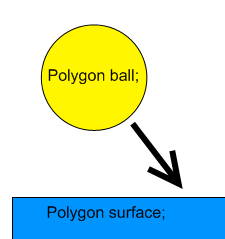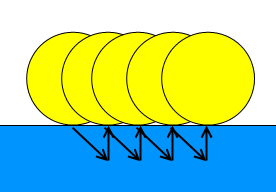FlatRedBall.Math.Geometry.Polygon.CollideAgainstMove

Introduction

The CollideAgainstMove is a method which can be used to test whether two Shapes are touching, and if they are to move one or both so that they no longer overlap. CollideAgainstMove also works between all types of shapes.

The CollideAgainstMove is a very common method used in games which include solid collision. Sometimes the collision is between two movable objects (such as a player and a box which can be pushed), and sometimes the collision is between a movable and static object (such as a player and a wall).

CollideAgainstMove values

CollideAgainstMove lets you specify how objects should behave when colliding. The first argument is the object to collide against, the second is the mass of the caller, and the third is the mass of the object colliding against.

For example, to collide a Player entity against a wall (assuming the entity implements ICollidable):

// Player has a mass of 0, wall has a mass of 1
PlayerInstance.CollideAgainstMove(WallCollision, 0, 1);

// If your Player object does not implement ICollidable, then you will need to use the shape on the Entity:
PlayerInstance.CollisionObject.CollideAgainstMove(WallCollision, 0, 1);

Inversely to have a player push a block, and have the player not slow down at all when pushing the block:

// Player has a mass of 1, wall has a mass of 0
PlayerInstance.CollideAgainstMove(BlockInstance, 1, 0);

To have both objects impacted equally by the collision, the same mass can be used. For example, if two cars collide (again assuming that the cars implement ICollidable)

CarInstance1.CollideAgainstMove(CarInstance2, 1, 1);

Any value can be used for the mass of the two objects – you’re not limited to using 0 and 1. We use 0 and 1 above to express intent – that one object should be mass-less, or that the two objects should have the same mass. For example, a heavier object could collide against a lighter object:

// In this case the truck's mass is 3 times as great as the car
HeavyTruckInstance.CollideAgainstMove(LightCarInstance, 3, 1);

Code Example

The following code loads a .plylstx to create a PositionedObjectList containing Polygons. Another Polygon is created which is controlled by the Keyboard. Move collision is used to keep the moving Polygon and the Polygons loaded from the .plylstx from overlapping. Notice that the mass variables can be modified to allow for different collision behavior.

Files Used: Smiley.plylstx

using FlatRedBall.Math;
using FlatRedBall.Math.Geometry;
using FlatRedBall.Content.Polygon;
using FlatRedBall.Input;

Add the following at class scope:

Polygon mControlledPolygon;

Add the following in Initialize after initializing FlatRedBall:

mControlledPolygon = Polygon.CreateEquilateral(6, 1, 0);
mControlledPolygon.Color = Microsoft.Xna.Framework.Graphics.Color.Orange;

PolygonSaveList polygonSaveList = PolygonSaveList.FromFile(@"Smiley.plylstx");

InputManager.Keyboard.ControlPositionedObject(mControlledPolygon);
{
mControlledPolygon.CollideAgainstMove(
polygon, 0, 1);
}Understanding the CollideAgainstMove Implementation

In brief mathematical terms, the CollideAgainstMove repositions the calling shape and the argument shape along the vector that is normal to the surface vector at the point of collision. The amount that each object moves when colliding depends on the two masses passed in to the method.

For example, consider the following situation. There are two Polygons – ball and surface. The ball has a positive XVelocity and negative YVelocity, causing it to fall down and to the right toward the surface Polygon:Eventually the ball will overlap the surface. Let’s assume that the following method is being called every frame:

ball.CollideAgainstMove(surface, 0, 1);

In this case the ball is given a 0 mass and the surface is given a 1 mass. In other words, surface will never be moved by this method while ball will.

When a collision occurs, the vector of the edge where the collision happened is determined. Then the normal (perpendicular) vector is calculated and the shape(s) is (are) moved along the normal vector the required distance to separate them. For example, the first time ball penetrates surface, the following edge vector and reposition vector are calculated:Since the ball has 0 mass, it will be moved by the full reposition vector. If the value were different, say .5 and .5, then ball and surface would each move half of the reposition vector. Of course, surface would move in the opposite direction as ball so that the two separate after the call.

Since this CollideAgainstMove resolves this penetration, it is never seen when the engine draws the shapes. But if the velocity of ball is not changed, then it will continue to penetrate surface every frame, then get pushed back out every frame. The result is what appears to be a smooth sliding movement across as follows:RepositionDirections

If one of the colliding shapes is an AxisAlignedRectangle then the direction of the “move” (the reposition) is subject to this value. For more information, see the RepositionDirections page.